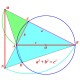## Finite and infinite regions defined by n lines in the plane

It is well known result in plane geometry: The maximum number of regions defined by n lines in the plane is R(n) = n(n+1)/2 +1. Of course, there are not any parallel pair from these lines. All sets of lines discussed here covers this future.

In these R(n) regions we can see some of them are finite and some of them are infinite (see figure). Please note that infinite region contains infinite ends of some lines. We denote number of finite regions as fR(n) and number of infinite regions as iR(n).

Of course R(n) = fR(n) + iR(n)

Now we try to calculate iR(n) and then fR(n).

Suppose now we have n lines with iR(n) infinite regions. We draw any new line L so we have now n+1 lines (see figure).

The line L has two infinite ends say A, B. From one this infinite end A we can see the line L divide one old infinite region (A) into two new infinite regions (1) and (2). So the number of infinite regions increases by 1. Similarly, from other infinite end B of L we see that the number of infinite regions increases by 1 also.

It means that iR(n+1) = iR(n) + 2

n = 1, iR(1) = 2

n = 2, iR(2) = 4

Therefor iR(n) = 2n

fR(n) = R(n) – iR(n) = n(n+1)/2 +1 – 2n = (n-1)(n-2)/2

Our conclude results:

1. The number of infinite regions defined by n lines is iR(n) = 2n
2. The maximum number of finite regions defined by n lines is fR(n) = (n-1)(n-2)/2